# ana3AIMEC: Aimec¶

ana3AIMEC (Automated Indicator based Model Evaluation and Comparison, [Fis13]) is a post-processing module to analyze and compare results from avalanche simulations. It enables the comparison of different simulations (with different input parameter sets for example, or from different models) of the same avalanche (going down the same avalanche path) in a standardized way. To do so, the ratser results are transformed into the path following coordinate system to make data comparable.

In AvaFrame/avaframe/runScripts, two different run scripts are provided and show examples on how the post-processing module ana3AIMEC can be used:

• full Aimec analysis for simulation results of one computational module (from 1 simulation to x simulations). runScripts.runAna3AIMEC.runAna3AIMEC()

• using Aimec to compare the results of two different computational modules (one reference for the reference computational module and multiple simulations in the other computation module). runScripts.runAna3AIMECCompMods.runAna3AIMECCompMods()

In all cases, one needs to provide a minimum amount of input data. Bellow is an example workflow for the full Aimec analysis, as provided in runScripts/runAna3AIMEC.py:

## Inputs¶

• raster of the DEM (.asc file)

• avalanche path in LINES (as a shapefile named NameOfAvalanche/Inputs/LINES/path_aimec.shp)

• a splitPoint in POINTS (as a shapefile named NameOfAvalanche/Inputs/POINTS/splitPoint.shp)

• Results from avalanche simulation (when using results from com1DFA, the helper function ana3AIMEC.dfa2Aimec.mainDfa2Aimec() in ana3AIMEC.dfa2Aimec fetches and prepares the input for Aimec)

• a method to define the reference simulation. By default, an arbitrary simulation is defined as reference. This can be changed in the ana3AIMEC/local_ana3AIMECCfg.ini as explained in Defining the reference simulation.

Note

The spatial resolution of the DEM and its extent can differ from the result raster data. Spatial resolution can also differ between simulations. If this is the case, the spatial resolution of the reference simulation results raster is used (default) or the resolution specified in the configuration file (cellSizeSL) is used if this one is provided. This way, all simulations will be transformed and analyzed using the same resolution.

## Outputs¶

• output figures in NameOfAvalanche/Outputs/ana3AIMEC/com1DFA/

• csv file with the results in NameOfAvalanche/Outputs/ana3AIMEC/com1DFA/ (a detailed list of the results is described in moduleAna3AIMEC.analyze-results)

## To run¶

• first go to AvaFrame/avaframe

• in your local copy of ana3AIMEC/ana3AIMECCfg.ini you can adjust the default settings (if not, the standard settings are used)

• enter path to the desired NameOfAvalanche/ folder in your local copy of avaframeCfg.ini

• run:

python3 runScripts/runAna3AIMEC.py


## Theory¶

AIMEC (Automated Indicator based Model Evaluation and Comparison, [Fis13]) was developed to analyze and compare avalanche simulations. The computational module presented here is inspired from the original AIMEC code. The simulations are analyzed and compared by projecting the results along a chosen poly-line (same line for all the simulations that are compared) called avalanche path. The raster data, initially located on a regular and uniform grid (with coordinates x and y) is projected on a regular non uniform grid (grid points are not uniformly spaced) that follows the avalanche path (with curvilinear coordinates (s,l)). This grid can then be “straightened” or “deskewed” in order to plot it in the (s,l) coordinates system.

The simulation results (two dimensional fields of e.g. peak velocities / pressure or flow thickness) are processed in a way that it is possible to compare characteristic values that are directly linked to the flow variables such as maximum peak flow thickness, maximum peak velocity or deduced quantities, for example maximum peak pressure, pressure based runout (including direct comparison to possible references, see Area indicators) for different simulations. The following figure illustrates the raster transformation process.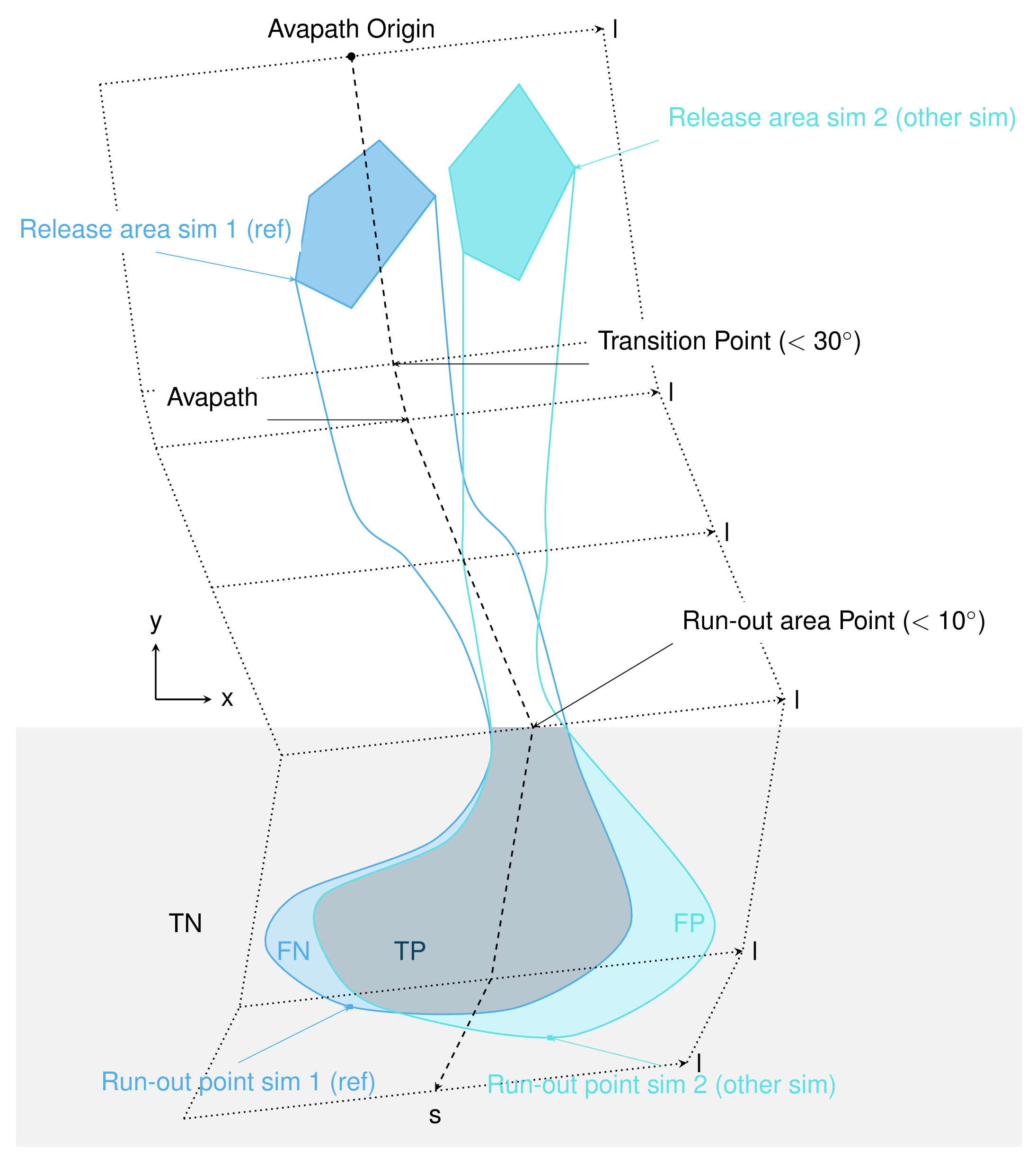Fig. 13 In the real coordinate system (x,y)¶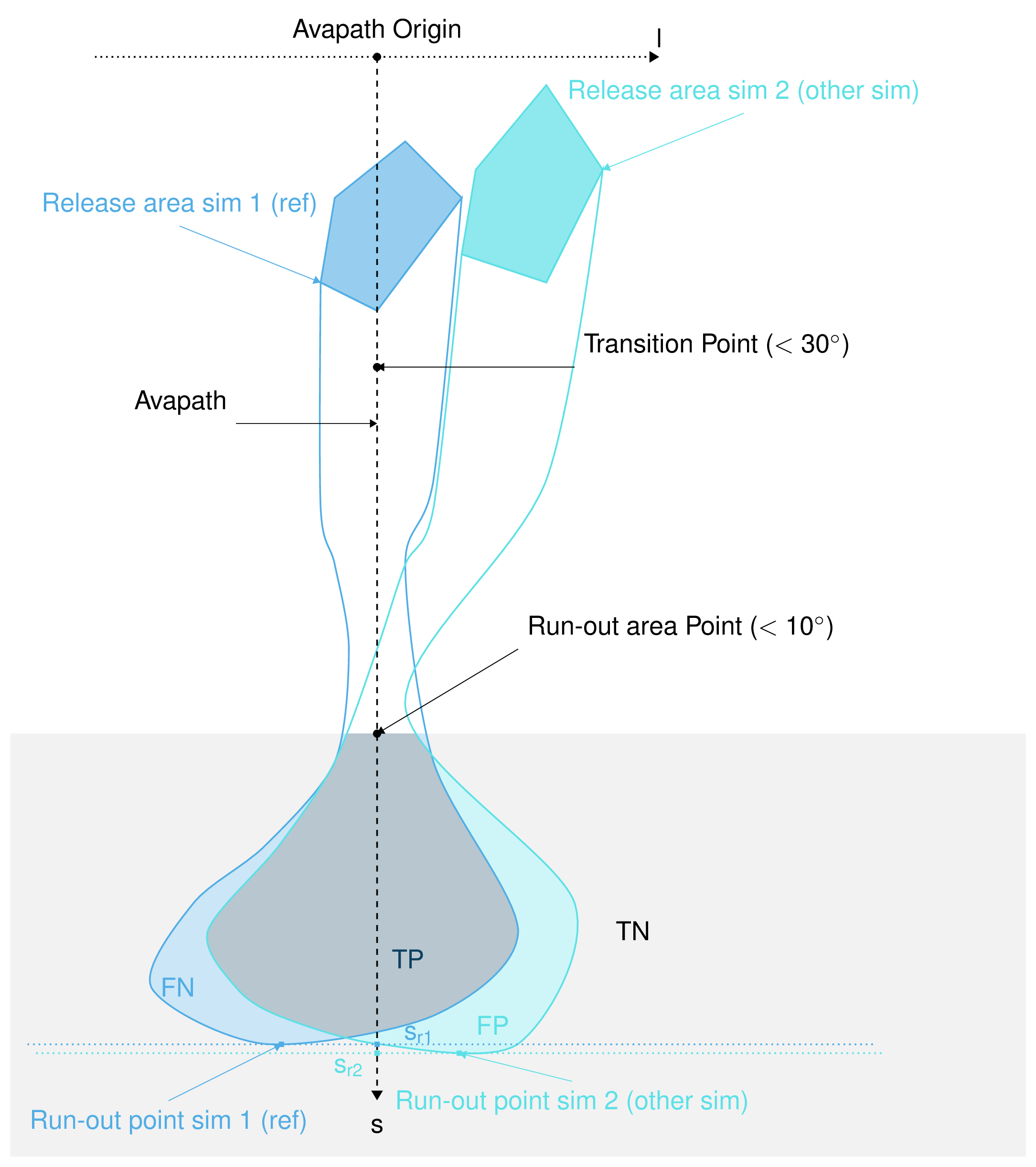Fig. 14 In the new coordinate system (s,l)¶

Here is the definition of the different indicators and outputs from the AIMEC post-processing process:

### Mean and max values along path¶

All two dimensional field results (for example peak velocities / pressure or flow thickness) can be projected into the curvilinear system using the previously described method. The maximum and average values of those fields are computed in each cross-section (l direction). For example the maximum $$A_{cross}^{max}(s)$$ and average $$\bar{A}_{cross}(s)$$ of the two dimensional distribution $$A(s,l)$$ is:

$A_{cross}^{max}(s) = \max_{\forall l \in [-\frac{w}{2},\frac{w}{2}]} A(s,l) \quad\mbox{and}\quad \bar{A}_{cross}(s) = \frac{1}{w}\int_{-\frac{w}{2}}^{\frac{w}{2}} A(s,l)dl$

### Runout point¶

The runout point is always given with respect to a peak result field ($$A(s,l)$$ which could be peak pressure or flow thickness, etc.) and a threshold value ($$A_{lim}>0$$). The runout point ($$s=s_{runout}$$) and the respective $$(x_{runout},y_{runout})$$ in the original coordinate system, correspond to the last point in flow direction where the chosen peak result $$A_{cross}^{max}(s)$$ is above the threshold value $$A_{lim}$$.

Note

It is very important to note that the position of the runout point depends on the chosen threshold value and peak result field. It is also possible to use $$\bar{A}_{cross}(s)>A_{lim}$$ instead of $$A_{cross}^{max}(s)>A_{lim}$$ to define the runout point.

### Runout length¶

This length depends on what is considered to be the beginning of the avalanche $$s=s_{start}$$. It can be related to the release area, to the transition point (first point where the slope angle is below $$30^{\circ}$$), to the runout area point (first point where the slope angle is below $$10^{\circ}$$) or in a similar way as $$s=s_{runout}$$ is defined saying that $$s=s_{start}$$ is the first point where $$A_{cross}^{max}(s)>A_{lim}$$ (this is the option implemented in ana3AIMEC.ana3AIMEC.py). The runout length is then defined as $$L=s_{runout}-s_{start}$$.

### Mean and max indicators¶

From the maximum values along path of the distribution $$A(s,l)$$ calculated in Mean and max values along path, it is possible to calculate the global maximum (MMA) and average maximum (AMA) values of the two dimensional distribution $$A(s,l)$$:

$MMA = \max_{\forall s \in [s_{start},s_{runout}]} A_{cross}^{max}(s) \quad\mbox{and}\quad AMA = \frac{1}{s_{runout}-s_{start}}\int_{s_{start}}^{s_{runout}} A_{cross}^{max}(s)ds$

### Area indicators¶

When comparing the runout area (corresponding to a given threshold $$A_{cross}^{max}(s)>A_{Lim}$$) of two simulations, it is possible to distinguish four different zones. For example, if the first simulation (sim1) is taken as reference and if True corresponds to the assertion that the avalanche reached this zone (reached means $$A_{cross}^{max}(s)>A_{Lim}$$) and False the avalanche did not reached this zone, those four zones are:

• TP (true positive) zone: green zone on Fig. 14 , sim1 = True sim2 = True

• FP (false positive) zone: blue zone on Fig. 14 , sim1 = False sim2 = True

• FN (false negative) zone: red zone on Fig. 14 , sim1 = True sim2 = False

• TN (true negative) zone: gray zone on Fig. 14 , sim1 = False sim2 = False

The two simulations are identical (in the runout zone) when the area of both FP and FN are zero. In order to provide a normalized number describing the difference between two simulations, the area of the different zones is normalized by the area of the reference simulation $$A_{ref} = A_{TP} + A_{FP}$$. This leads to the 4 area indicators:

• $$\alpha_{TP} = A_{TP}/A_{ref}$$, which is 1 if sim2 covers at least the reference

• $$\alpha_{FP} = A_{FP}/A_{ref}$$, which is a positive value if sim2 covers an area outside of the reference

• $$\alpha_{FN} = A_{FN}/A_{ref}$$, which is a positive value if the reference covers an area outside of sim2

• $$\alpha_{TN} = A_{TN}/A_{ref}$$ (this value may not be of great interest because it depends on the width and length of the entire domain of the result rasters (s,l))

Identical simulations (in the runout zone) lead to $$\alpha_{TP} = 1$$ , $$\alpha_{FP} = 0$$ and $$\alpha_{FN} = 0$$

### Mass indicators¶

From the analysis of the release mass ($$m_r$$ at the beginning, i.e $$t = t_{ini}$$), total mass ($$m_t$$ at the end, i.e $$t = t_{end}$$) and entrained mass ($$m_e$$ at the end, i.e $$t = t_{end}$$) it is possible to calculate the growth index $$GI$$ and growth gradient $$GG$$ of the avalanche:

$GI = \frac{m_t}{m_r} = \frac{m_r + m_e}{m_r} \quad\mbox{and} \quad GG = \frac{m_r + m_e}{t_{end}-t_{ini}}$

Time evolution of the total mass and entrained one are also analyzed.

## Procedure¶

This section describes how the theory is implemented in the ana3AIMEC module.

### Defining the reference simulation¶

To apply a complete Aimec analysis, a reference simulation needs to be defined. The analysis of the other simulations will be compared to the one of the reference simulation. The reference simulation can be determined by its name (or part of the name) or based on some configuration parameter and value (to adjust in the local copy of ana3AIMEC/ana3AIMECCfg.ini) if it comes from the com1DFA module (or any computational module that provides a configuration).:

• Based on the simulation name

one needs to provide a not-empty string in the AIMEC configuration file for the referenceSimName parameter. This string can be a part or the full name of the reference simulation. A warning is raised if several simulation match the criterion (can happen if part of the name is given) and the first simulation found is arbitrarily taken as reference.

• Based on some configuration parameter

one needs to provide a varParList (parameter or list of parameters separated by |) in the AIMEC configuration file as well as the desired sorting order (ascendingOrder, True by default) for these parameters and optionally a referenceSimValue. The simulations are first going to be sorted according to varParList and ascendingOrder (this is done by the pandas function sort_values). The reference simulation is either the first simulation found after sorting if no referenceSimValue or the simulation matching the referenceSimValue provided (closest value if the parameter is a float or integer, case insensitive for strings). If multiple simulations match the criterion, the first simulation is taken as reference and a warning is raised.

### Perform path-domain transformation¶

First, the transformation from (x,y) coordinate system (where the original rasters lie in) to (s,l) coordinate system is applied given a new domain width (domainWidth). This is done by ana3AIMEC.aimecTools.makeDomainTransfo(). A new grid corresponding to the new domain (following the avalanche path) is built with a cell size defined by the reference simulation (default) or cellSizeSL if provided. The transformation information are stored in a rasterTransfo dictionary (see ana3AIMEC.aimecTools.makeDomainTransfo() for more details).

### Assign data¶

The simulation results (for example peak velocities / pressure or flow thickness) are projected on the new grid using the transformation information by ana3AIMEC.aimecTools.assignData(). The projected results are stored in the newRasters dictionary.

This results in the following plot: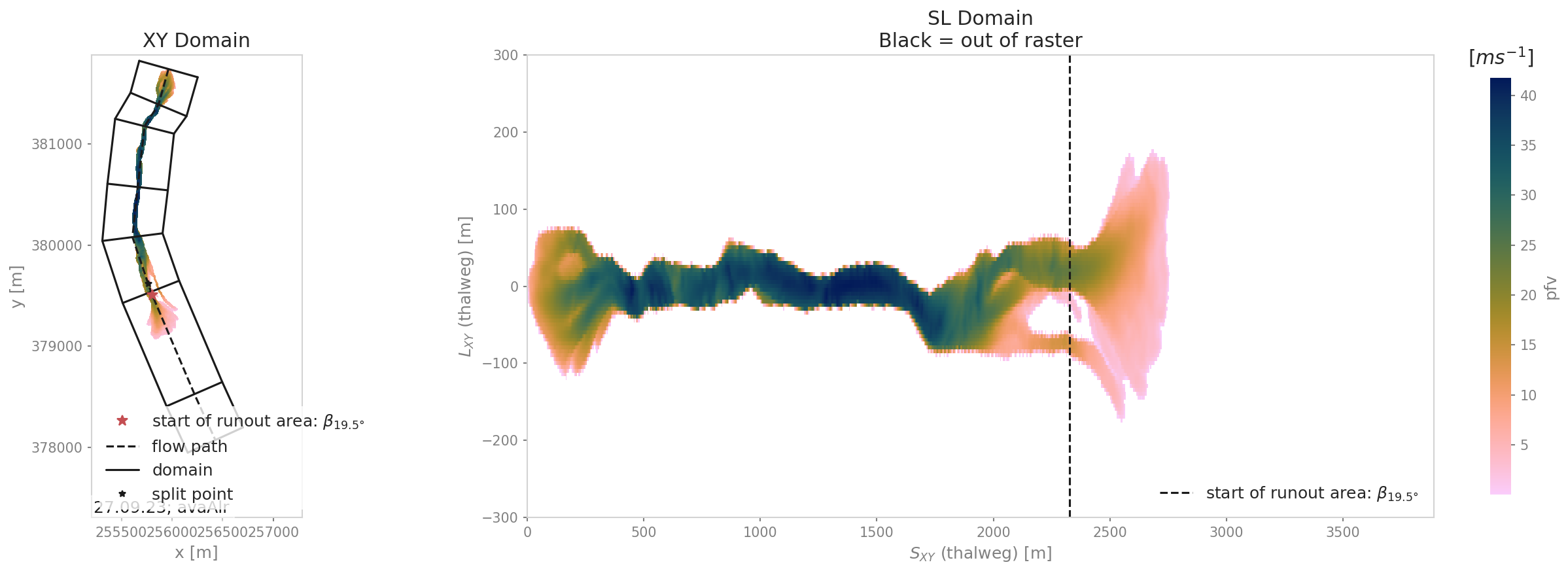Fig. 15 Alr avalanche coordinate transformation and peak pressure field reprojetion.

### Analyze results¶

Calculates the different indicators described in the Theory section for a given threshold. The threshold can be based on pressure, flow thickness, … (this needs to be specified in the configuration file). Returns a resAnalysisDF dataFrame with the analysis results (see ana3AIMEC.ana3AIMEC.postProcessAIMEC() for more details). In this dataFrame there are multiple columns, one for each result from the analysis (one column for runout length, one for MMA, MAM…) and one row for each simulation analyzed.

### Plot and save results¶

Plots and saves the desired figuresand writes results in resAnalysisDF to a csv file. By default, Aimec saves five summary plots plus three plots per simulation comparing the numerical simulations to the reference. The five summary plots are :

• “DomainTransformation” shows the real domain on the left and new domain on the right (Fig. 15)

• “referenceFields” shows the peak pressure, flow thickness and velocity in the new domain

• “slComparison” shows the difference between all simulations in terms of peak values along profile. If only two simulations are provided, a three panel plot like the following is produced: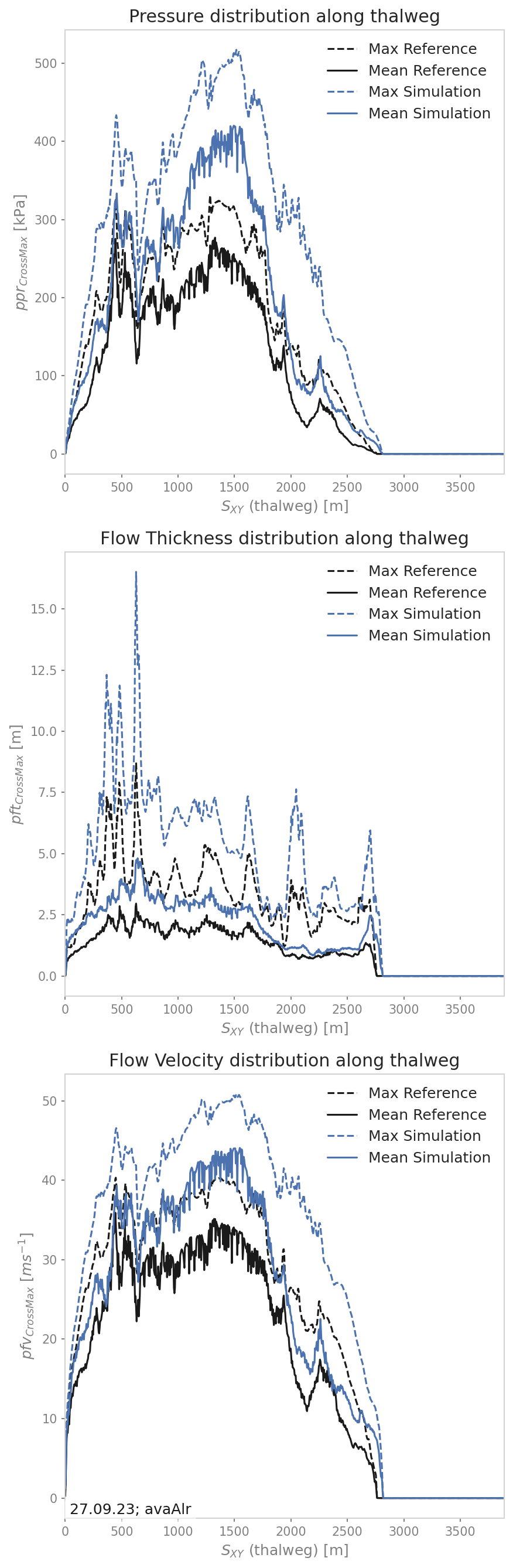Fig. 17 Maximum peak fields comparison between two simulations

if more than two simulations are provided only the peak field specified in the configuration file is analyzed and the statistics in terms of peak value along profile are plotted (mean, max and quantiles):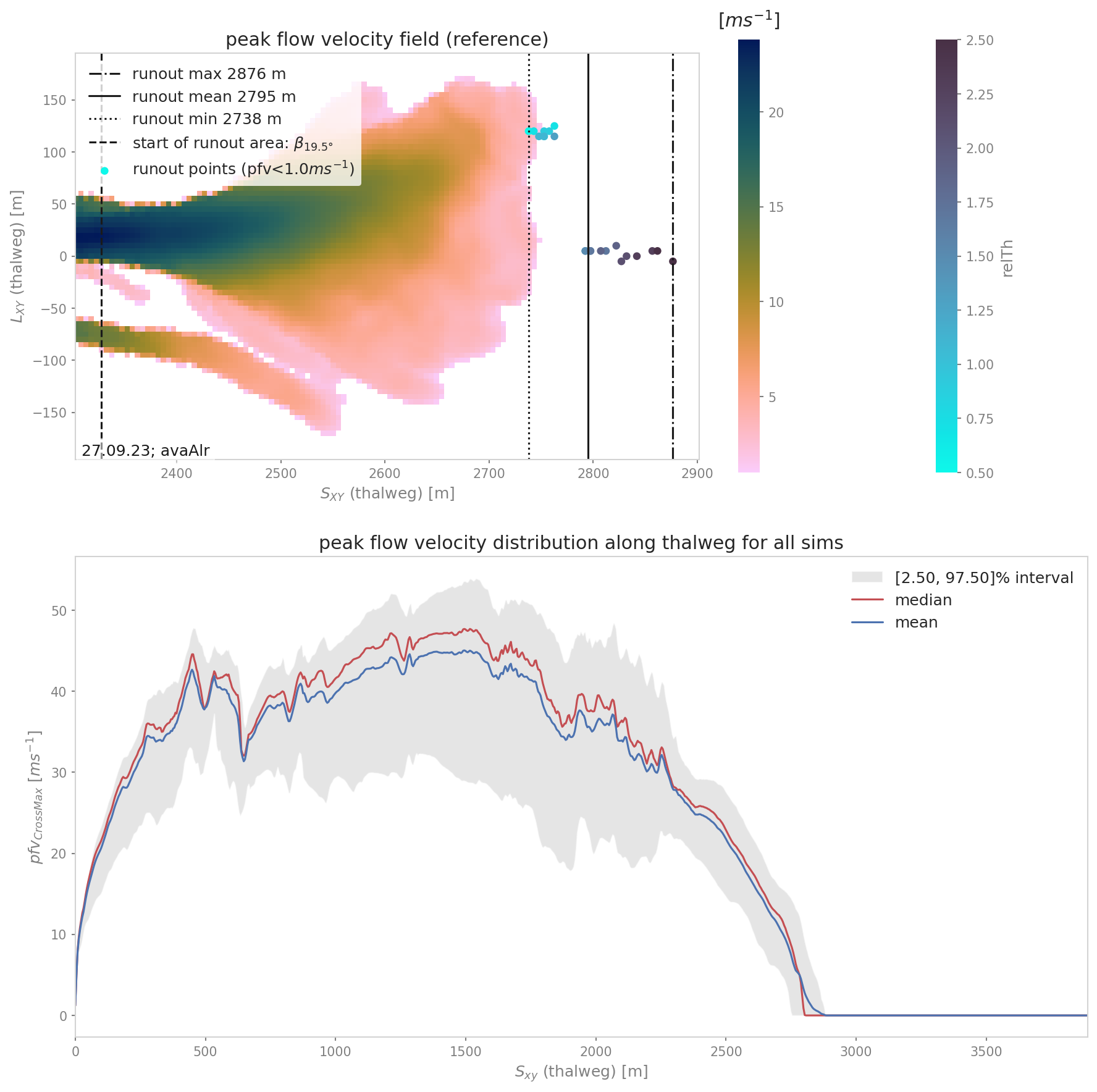Fig. 18 Maximum peak pressure distribution along path

• “ROC” shows the normalized area difference between reference and other simulations.

• “relMaxPeakField” shows the relative difference in maximum peak value between reference and other simulation function of runout lengthFig. 20 Relative maximum peak pressure function of runout

The last plots “_hashID_ContourComparisonToReference”, “_hashID_AreaComparisonToReference” and “_hashID_massAnalysis” where “hashID” is the name of the simulation show the 2D difference to the reference, the statistics associated and the mass analysis figure (this means these figures are created for each simulation).Fig. 21 The area comparison plot shows the false negative (FN in blue, which is where the reference field exceeds the threshold but not the simulation) and true positive (TP in red, which is where the simulation field exceeds the threshold but not the reference) areas. and reference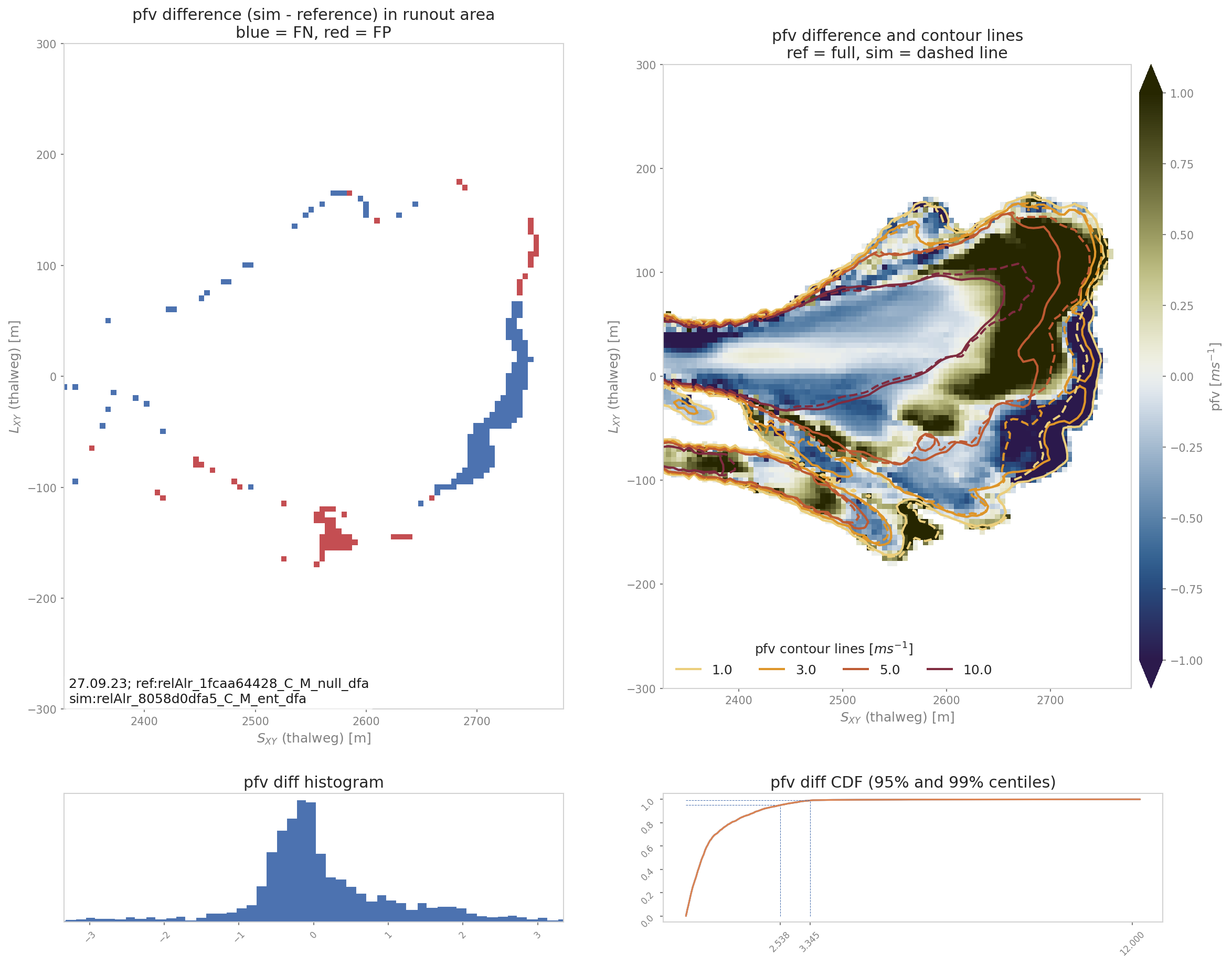Fig. 22 The contour comparison plot shows the contour lines of the reference (full lines) and the simulation (dashed lines) of the desired result fields in the runout area. It also shows the difference between the reference and simulation and computes the repatriation of this difference (Probability Density Function and Cumulative Density Function of the difference)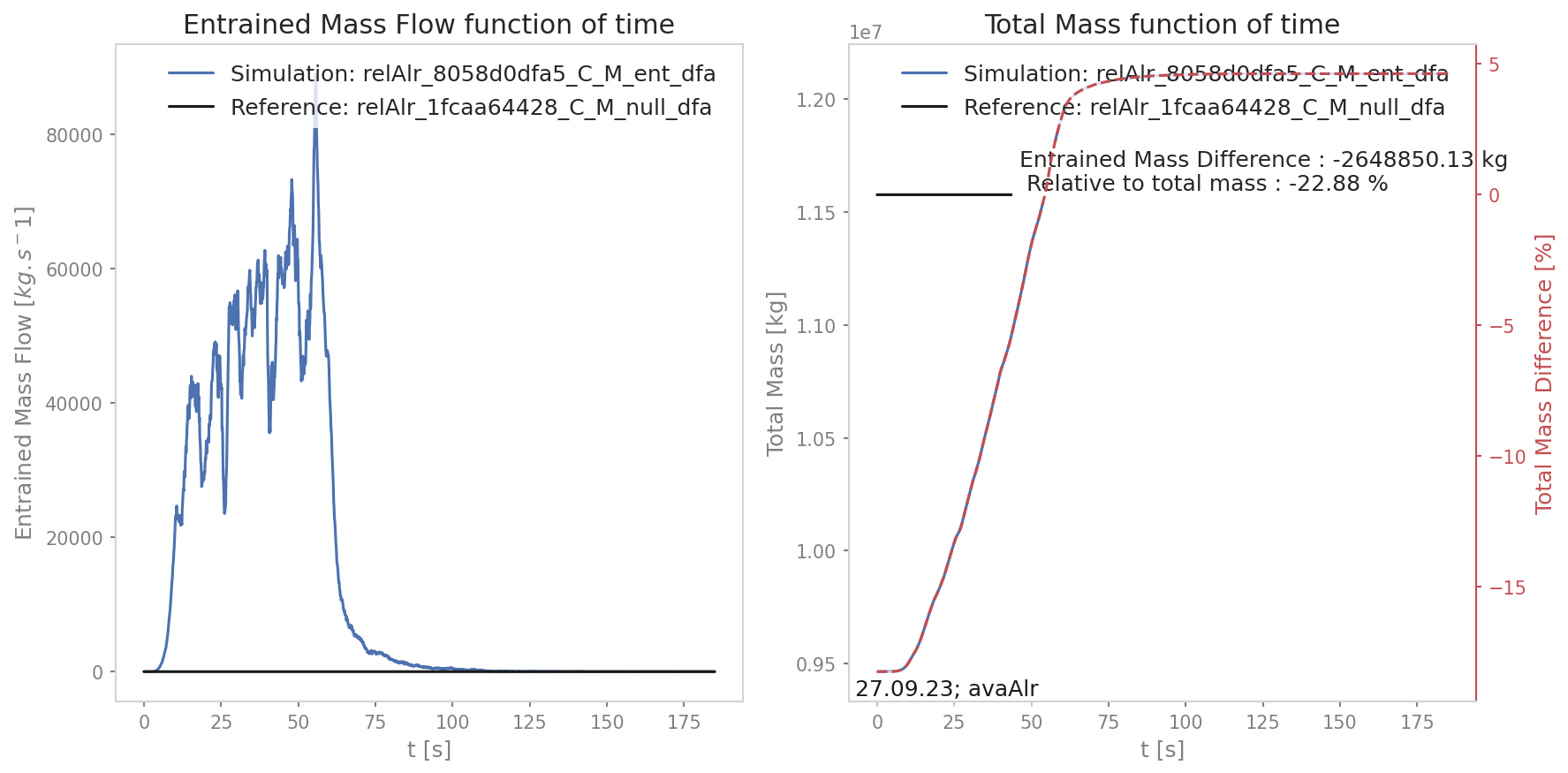Fig. 23 The mass analysis plot shows the evolution of the total and entrained mass during the simulation and compares it to the reference

## Configuration parameters¶

All configuration parameters are explained in ana3AIMEC/ana3AIMECCfg.ini (and can be modified in a local copy ana3AIMEC/local_ana3AIMECCfg.ini):

### Config File - This file contains the main settings for the ana3AIMEC run
# This file is part of Avaframe.
# This file will be overridden by local_ana3AIMEC.ini if it exists

# Optional settings-------------------------------
[AIMECSETUP]
# width of the domain around the avalanche path in [m]
domainWidth = 600

# The cell size for the new (s,l) raster is automatically computed from the reference result file (leave the following field empty)
# It is possible to force the cell size to take another value, then specify this new value below, otherwise leave empty (default).
cellSizeSL =

# angle for the start of the run-out zone
startOfRunoutAreaAngle = 10

# data result type for general analysis (ppr|pft|pfd). If left empty takes the result types available for all simulations
resTypes = ppr|pft|pfv

# data result type for runout analysis (ppr, pft, pfv)
runoutResType = ppr

# limit value for evaluation of runout (depends on the runoutResType chosen)
thresholdValue = 1

# contour levels value for the difference plot (depends on the runoutResType chosen)
# use | delimiter (for ppr 1|3|5|10, for pft 0.1|0.25|0.5|0.75|1)
contourLevels = 1|3|5|10

# max of runoutResType difference for contour lines plus capped difference in runoutResType plot (for example 1 (pft), 5 (ppr))
diffLim = 1

# percentile to display when analyzing the peak values along profile
# for example 5 (corresponding to 5%) will lead to the interval [2.5, 97.5]%
percentile = 5
# chose interpolation method between 'nearest' and 'bilinear'
interpMethod = bilinear

# threshold distance [m]. When looking for the beta point make sure at least
# dsMin meters after the beta point also have an angle bellow 10°
dsMin = 30

# computational module that was used to produce avalanche simulations (to locate peakFiles)
anaMod = com1DFA

# two computational module that were used to produce avalanche simulations (to locate peakFiles) for comparison separated by |
comModules =

# if a computation module is benchmark, specify the test name (we assume that the testName folder is in AvaFrame/benchmarks/)
testName =

# parameter used for ordering the simulations - multiple possible; (e.g.relTh|deltaTh)
varParList =
# True if ascending ordering of simulations for varPar, False if descending order
ascendingOrder = True
# parameter value (first parameter in varParList) that should be used as reference simulation (e.g. 1.0)
referenceSimValue =
# OR directly set reference simulation by its name (name of simulation result file or parts of it that definitively
# identify one particular simulation)
referenceSimName =
# unit of desired result parameter (e.g. m)
unit =

#---------------------------------------

# plot save results flags------------------------
[FLAGS]
# Which value to plot in resultVisu
# 1 = mean pressure data
# 2 = growth index
# 3 = max pressure data
typeFlag = 3
# number of simulations above which a (additional) density plot is created
nDensityPlot = 100

# Mass analysis
flagMass = True

#----------------------------------------------------------